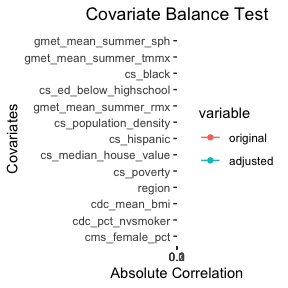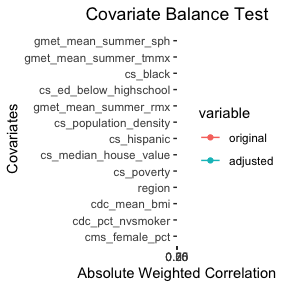# Synthetic Medicare Data

library(CausalGPS)
library(ggplot2)

In this vignette, we present application of CausalGPS package on the Synthetic Medicare Data. In the dataset, we link the 2010 synthetic Medicare claims data to environmental exposures and potential confounders. The dataset is hosted on Harvard Dataverse (Khoshnevis, Wu, and Braun 2022).

data("synthetic_us_2010")
data <- synthetic_us_2010
knitr::kable(head((data)))
FIPS qd_mean_pm25 NAME cs_poverty cs_hispanic cs_black cs_white cs_native cs_asian cs_ed_below_highschool cs_household_income cs_median_house_value cs_total_population cs_other cs_area cs_population_density cdc_mean_bmi cdc_pct_cusmoker cdc_pct_sdsmoker cdc_pct_fmsmoker cdc_pct_nvsmoker cdc_pct_nnsmoker gmet_mean_tmmn gmet_mean_summer_tmmn gmet_mean_winter_tmmn gmet_mean_tmmx gmet_mean_summer_tmmx gmet_mean_winter_tmmx gmet_mean_rmn gmet_mean_summer_rmn gmet_mean_winter_rmn gmet_mean_rmx gmet_mean_summer_rmx gmet_mean_winter_rmx gmet_mean_sph gmet_mean_summer_sph gmet_mean_winter_sph cms_mortality_pct cms_white_pct cms_black_pct cms_others_pct cms_hispanic_pct cms_female_pct STATE STATE_CODE region
1001 11.85557 Autauga County, Alabama 0.0858086 0.0028053 0.1320132 0.8524752 0.0087459 0.0026403 0.2533003 37351 133900 53155 0.0013201 594.436 89.42090 3208.390 0.1463415 0.0243902 0.2439024 0.5609756 0.0243902 283.8634 295.4464 273.0409 297.2615 307.2943 284.5251 40.42980 45.25372 43.95282 88.97211 95.95946 85.72278 0.0094171 0.0167348 0.0040017 0.0000000 0.5958904 0.4041096 0.0000000 0.0000000 0.5547945 1 AL SOUTH
1003 10.43793 Baldwin County, Alabama 0.0533287 0.0140393 0.0515350 0.9186271 0.0054157 0.0022422 0.1609521 40104 177200 175791 0.0081407 1589.784 110.57540 3249.755 0.1470588 0.0392157 0.2450980 0.5686275 0.0000000 285.8735 296.6723 275.4020 298.1579 306.4516 287.3572 42.34226 50.02479 43.42646 90.28333 95.14939 88.16227 0.0102607 0.0172820 0.0047839 0.0227273 0.6384298 0.3533058 0.0082645 0.0000000 0.5392562 1 AL SOUTH
1005 11.50424 Barbour County, Alabama 0.1944298 0.0094587 0.3334209 0.6560694 0.0000000 0.0000000 0.4051498 22143 88200 27699 0.0010510 884.876 31.30269 2953.693 0.0714047 0.0504250 0.2168344 0.5645023 0.0111988 284.1352 295.3782 273.8296 297.6494 307.0302 285.5848 40.05212 46.02080 43.77853 90.76408 96.72028 86.72719 0.0097046 0.0169258 0.0043431 0.0000000 0.6901408 0.2816901 0.0281690 0.0000000 0.5070423 1 AL SOUTH
1007 11.88692 Bibb County, Alabama 0.1130868 0.0010669 0.1219772 0.8662873 0.0000000 0.0000000 0.3886913 24875 81200 22610 0.0106686 622.582 36.31650 3255.287 0.1034483 0.0229885 0.3103448 0.5517241 0.0114943 283.5388 295.3145 272.5781 296.7969 307.0417 283.9070 41.59857 47.24975 43.87743 89.57623 96.48472 85.84614 0.0093942 0.0169568 0.0038307 0.0215264 0.6555773 0.3405088 0.0039139 0.0000000 0.5714286 1 AL SOUTH
1009 11.65920 Blount County, Alabama 0.1047926 0.0094363 0.0105538 0.9684629 0.0058356 0.0000000 0.3594487 25857 113700 56692 0.0057114 644.776 87.92511 3500.333 0.0833333 0.0000000 0.2500000 0.6388889 0.0277778 282.6099 294.7041 271.3427 295.5558 306.4517 281.9145 42.44578 48.28317 46.07121 88.72523 96.37532 84.95932 0.0089541 0.0165894 0.0035027 0.0062112 0.7018634 0.2981366 0.0000000 0.0000000 0.6086957 1 AL SOUTH
1011 11.65386 Bullock County, Alabama 0.1701807 0.0000000 0.5903614 0.4096386 0.0000000 0.0000000 0.4487952 20500 66300 10923 0.0000000 622.805 17.53839 3400.474 0.1282051 0.0384615 0.3333333 0.5000000 0.0000000 283.3906 295.0217 273.1478 297.2145 306.8042 285.0072 41.29272 46.77274 44.80368 92.81798 97.60276 88.14277 0.0096621 0.0169198 0.0042435 0.0103245 0.6666667 0.3185841 0.0132743 0.0014749 0.5501475 1 AL SOUTH
# transformers
pow2 <- function(x) {x^2}
pow3 <- function(x) {x^3}
clog <- function(x) log(x+0.001)

confounders <- names(data)
confounders <- confounders[!(confounders %in% c("FIPS","Name","STATE",
"STATE_CODE","cms_mortality_pct",
"qd_mean_pm25"))]

## Examples of Generating Pseudo Population

### Scenario 1

• Causal Inference: Matching
• GPS model: Parametric
• Optimized_compile: True

confounders_s1 <- c("cs_poverty","cs_hispanic",
"cs_black",
"cs_ed_below_highschool",
"cs_median_house_value",
"cs_population_density",
"cdc_mean_bmi","cdc_pct_nvsmoker",
"gmet_mean_summer_tmmx",
"gmet_mean_summer_rmx",
"gmet_mean_summer_sph",
"cms_female_pct", "region"
)

study_data <- data[, c("qd_mean_pm25", confounders, "cms_mortality_pct")]
study_data$id <- seq_along(1:nrow(study_data)) study_data$region <- as.factor(study_data$region) study_data$cs_PIR <- study_data$cs_median_house_value/study_data$cs_household_income

# Choose subset of data
q1 <- stats::quantile(study_data$qd_mean_pm25, 0.25) q2 <- stats::quantile(study_data$qd_mean_pm25, 0.99)
trimmed_data <- subset(study_data[stats::complete.cases(study_data) ,],
qd_mean_pm25 <= q2  & qd_mean_pm25 >= q1)

trimmed_data$gmet_mean_summer_sph <- pow2(trimmed_data$gmet_mean_summer_sph)

set.seed(172)
pseudo_pop_1 <- generate_pseudo_pop(trimmed_data[, c("id",
"cms_mortality_pct")],
trimmed_data[, c("id",
"qd_mean_pm25")],
trimmed_data[, c("id",
confounders_s1)],
ci_appr = "matching",
gps_density = "normal",
bin_seq = NULL,
exposure_trim_qtls = c(0.0 ,
1.0),
use_cov_transform = TRUE,
transformers = list("pow2","pow3","clog"),
sl_lib = c("m_xgboost"),
params = list(xgb_nrounds=c(17),
xgb_eta=c(0.28)),
covar_bl_method = "absolute",
covar_bl_trs = 0.1,
covar_bl_trs_type = "mean",
max_attempt = 1,
dist_measure = "l1",
delta_n = 0.1,
scale = 1)
#> mean absolute correlation: 0.150596118336646| Covariate balance threshold: 0.1
#> mean absolute correlation: 0.046627261136326| Covariate balance threshold: 0.1
#> Covariate balance condition has been met (iteration: 1/1)
#> Best mean absolute correlation: 0.046627261136326| Covariate balance threshold: 0.1

plot(pseudo_pop_1)### Scenario 2

• Causal Inference: Matching
• GPS model: Parametric
• Optimized_compile: False
set.seed(172)
pseudo_pop_2 <- generate_pseudo_pop(trimmed_data[, c("id",
"cms_mortality_pct")],
trimmed_data[, c("id",
"qd_mean_pm25")],
trimmed_data[, c("id", confounders_s1)],
ci_appr = "matching",
gps_density = "normal",
bin_seq = NULL,
exposure_trim_qtls = c(0.0 ,
1.0),
use_cov_transform = TRUE,
transformers = list("pow2","pow3","clog"),
sl_lib = c("m_xgboost"),
params = list(xgb_nrounds=c(17),
xgb_eta=c(0.28)),
covar_bl_method = "absolute",
covar_bl_trs = 0.1,
covar_bl_trs_type = "mean",
max_attempt = 1,
dist_measure = "l1",
delta_n = 0.1,
scale = 1)
#> mean absolute correlation: 0.150596118336646| Covariate balance threshold: 0.1
#> mean absolute correlation: 0.046627261136326| Covariate balance threshold: 0.1
#> Covariate balance condition has been met (iteration: 1/1)
#> Best mean absolute correlation: 0.046627261136326| Covariate balance threshold: 0.1

plot(pseudo_pop_2)optimized_data_1 <- pseudo_pop_1$pseudo_pop[,c("qd_mean_pm25","gps","counter_weight")] nonoptimized_data_2 <- pseudo_pop_2$pseudo_pop[,c("qd_mean_pm25","gps","counter_weight")]

print(paste("Number of rows of data in the optimized approach: ",
nrow(optimized_data_1)))
#>  "Number of rows of data in the optimized approach:  2300"

print(paste("Number of rows of data in the non-optimized approach: ",
nrow(nonoptimized_data_2)))
#>  "Number of rows of data in the non-optimized approach:  2300"

print(paste("Sum of data samples in the optimized approach: ",
sum(optimized_data_1$counter_weight))) #>  "Sum of data samples in the optimized approach: 140300" print(paste("Number of data in the non-optimized approach: ", length(nonoptimized_data_2$qd_mean_pm25)))
#>  "Number of data in the non-optimized approach:  2300"

# Replicate gps values of optimized approach
expanded_opt_data_1 <- optimized_data_1[rep(seq_len(nrow(optimized_data_1)),
optimized_data_1$counter_weight), 1:3] exp_gps_a_1 <- expanded_opt_data_1$gps
gps_b_1 <- nonoptimized_data_2$gps differences <- sort(gps_b_1) - sort(exp_gps_a_1) print(paste("Sum of differences in gps values between optimized and ", "non-optimized approaches is: ", sum(differences))) #>  "Sum of differences in gps values between optimized and non-optimized approaches is: 38958.9033869061" ### Scenario 3 • Causal Inference: Matching • GPS model: Non-Parametric • Optimized_compile: True trimmed_data <- subset(study_data[stats::complete.cases(study_data) ,], qd_mean_pm25 <= q2 & qd_mean_pm25 >= q1) set.seed(8967) pseudo_pop_3 <- generate_pseudo_pop(trimmed_data[, c("id", "cms_mortality_pct")], trimmed_data[, c("id", "qd_mean_pm25")], trimmed_data[, c("id", confounders_s1)], ci_appr = "matching", gps_density = "kernel", bin_seq = NULL, exposure_trim_qtls = c(0.0 , 1.0), use_cov_transform = TRUE, transformers = list("pow2","pow3","clog"), sl_lib = c("m_xgboost"), params = list(xgb_nrounds=c(12), xgb_eta=c(0.1)), nthread = 1, covar_bl_method = "absolute", covar_bl_trs = 0.1, covar_bl_trs_type = "mean", max_attempt = 1, dist_measure = "l1", delta_n = 0.1, scale = 1) #> mean absolute correlation: 0.150596118336646| Covariate balance threshold: 0.1 #> mean absolute correlation: 0.0521458538948437| Covariate balance threshold: 0.1 #> Covariate balance condition has been met (iteration: 1/1) #> Best mean absolute correlation: 0.0521458538948437| Covariate balance threshold: 0.1 plot(pseudo_pop_3)### Scenario 4 • Causal Inference: Weighting • GPS model: Parametric • Optimized_compile: N/A trimmed_data <- subset(study_data[stats::complete.cases(study_data) ,], qd_mean_pm25 <= q2 & qd_mean_pm25 >= q1) trimmed_data$cs_poverty <- pow2(trimmed_data\$cs_poverty)

set.seed(672)
pseudo_pop_4 <- generate_pseudo_pop(trimmed_data[, c("id",
"cms_mortality_pct")],
trimmed_data[, c("id",
"qd_mean_pm25")],
trimmed_data[, c("id", confounders_s1)],
ci_appr = "weighting",
gps_density = "normal",
bin_seq = NULL,
exposure_trim_qtls = c(0.0 ,
1.0),
use_cov_transform = TRUE,
transformers = list("pow2","pow3","clog"),
sl_lib = c("m_xgboost"),
params = list(xgb_nrounds=c(35),
xgb_eta=c(0.14)),
covar_bl_method = "absolute",
covar_bl_trs = 0.1,
covar_bl_trs_type = "mean",
max_attempt = 1,
dist_measure = "l1",
delta_n = 0.1,
scale = 1)
#> mean absolute correlation: 0.150596118336646| Covariate balance threshold: 0.1
#> mean absolute correlation: 0.532572233294604| Covariate balance threshold: 0.1
#> Covariate balance condition has not been met.
#> Best mean absolute correlation: 0.532572233294604| Covariate balance threshold: 0.1

plot(pseudo_pop_4)## Covariate Balance

In the previous examples, we passed specific parameters for estimating GPS values. Achieving acceptable covariate balance can be computed by searching for the most appropriate parameters and might not be a simple task. This package uses transformers on the features to get an acceptable covariate balance. The following parameters are directly related to searching for an acceptable covariate balance.

• covar_bl_trs: Is the acceptable threshold to stop searching. It can be computed either by mean, median, or maximal value of features correlation, which is defined in covar_bl_trs_type.
• params: In different iterations, we choose a parameter at random from the provided list. For example, by xgb_nrounds=seq(1,100) in the parameters, nround parameter for xgboost trainer will be selected a number between 1 and 100 at random, at each iteration.
• transformers: After each iteration, we choose a feature with the highest correlation and apply a transformer from the provided list. All transformers should be applied to a feature before reapplying the same transformer on the same feature.
• max_attempt: Number of test iteration. If the covar_bl_trs is not met, the search will stop after max_attempt iteration and will return the best found population.

### Scenario 5

• Causal Inference: Matching + searching for acceptable covariate balance

• GPS model: Non-Parametric

• Optimized_compile: True

• Search domain:

• transformers: pow2, pow3, clog.
• nround for xgboost: 10-100.
• eta for xgboost: 0.1-0.5.
• max_attempt: 5.
• covar_bl_trs: 0.08.
• covar_bl_trs_type: mean
trimmed_data <- subset(study_data[stats::complete.cases(study_data) ,],
qd_mean_pm25 <= q2  & qd_mean_pm25 >= q1)

set.seed(328)
pseudo_pop_5 <- generate_pseudo_pop(trimmed_data[, c("id",
"cms_mortality_pct")],
trimmed_data[, c("id",
"qd_mean_pm25")],
trimmed_data[, c("id", confounders_s1)],
ci_appr = "matching",
gps_density = "kernel",
bin_seq = NULL,
exposure_trim_qtls = c(0.0 ,
1.0),
use_cov_transform = TRUE,
transformers = list("pow2","pow3","clog"),
sl_lib = c("m_xgboost"),
params = list(xgb_nrounds=seq(10, 100, 1),
xgb_eta=seq(0.1,0.5,0.01)),
covar_bl_method = "absolute",
covar_bl_trs = 0.08,
covar_bl_trs_type = "mean",
max_attempt = 5,
dist_measure = "l1",
delta_n = 0.1,
scale = 1)
#> mean absolute correlation: 0.150596118336646| Covariate balance threshold: 0.08
#> mean absolute correlation: 0.123983603260004| Covariate balance threshold: 0.08
#> mean absolute correlation: 0.20034674112753| Covariate balance threshold: 0.08
#> mean absolute correlation: 0.165910759386322| Covariate balance threshold: 0.08
#> mean absolute correlation: 0.160872521798447| Covariate balance threshold: 0.08
#> mean absolute correlation: 0.180468747869532| Covariate balance threshold: 0.08
#> Covariate balance condition has not been met.
#> Best mean absolute correlation: 0.123983603260004| Covariate balance threshold: 0.08

plot(pseudo_pop_5)In this example, after 5 attempts, we could not find a pseudo population that can satisfy the covariate balance test.